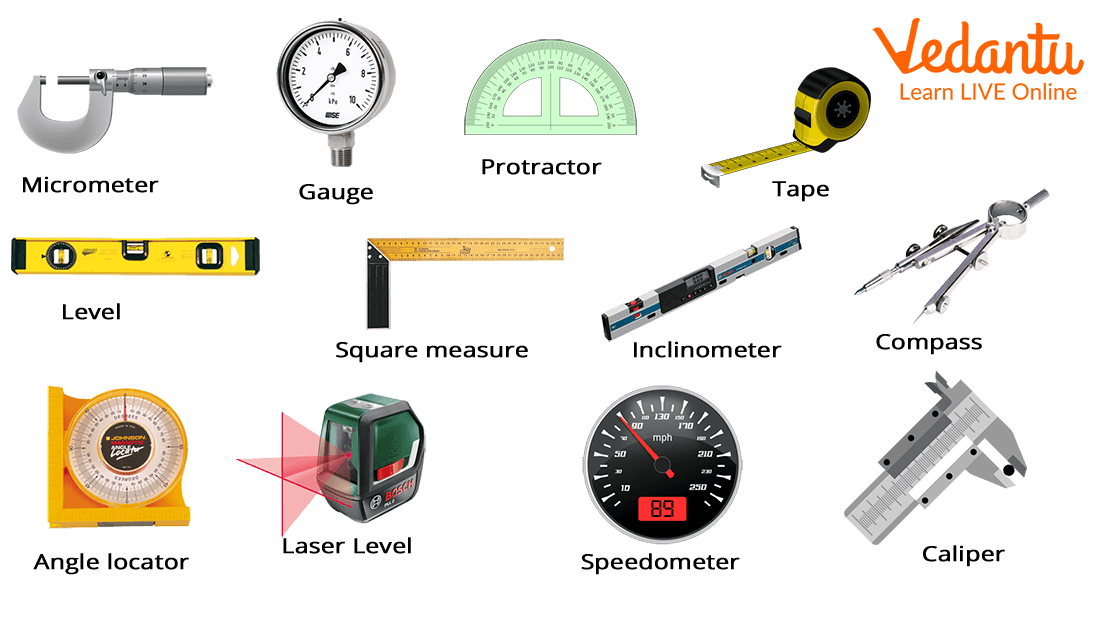Courses
Courses for Kids
Free study material
Free LIVE classes
More

# Measure## What is Measure?

Last updated date: 26th Jan 2023
Total views: 67.2k
Views today: 0.60k

Is milk the secret of your energy? Then, how many glasses of milk do you drink in a day? Two glasses, one in the morning and one at night. Good, that's like a champion.

Wait, what are you saying? You drink milk reluctantly. And, you are annoyed as we ask a question related to milk. My dear child, here are two points you have to remember:

1. Milk is good for your health.

2. ‘Two glass’ is the “measure” of milk.

The question we have asked is to tell you what is called ‘measure’? To know more, read this complete article.

## Definition of Measure

In general, the measure is the characteristic of any object, person, or activity in words, symbols, or units. 'Measure' is to find the height, weight, length, volume, and other parameters of any object.

For example: How old are you? Your answer probably is 6 or 7 years.

Here, the question is about your age. The measure is the characteristics that we obtain, here we obtain age. So, the measure is ‘age’.

To measure any characteristic, it is not necessary to have a defined unit.

For example: How much do you walk today? Two rounds of a park. So, here walking distance can be measured as two rounds of the park, which is not a unit in any defined format. But it is self-explanatory and used in day-to-day life.

A process to understand the characteristics of any object, person, or activity defined by using any standard units, symbols, or standard words is called ‘measure’.Measurement Devices

### Factors of ‘Measure’

There are four factors to ‘measure’ any object, which are the following:

1. Object, Person, or Activity: For which or whom ‘measure’ is required.

For example:

1. What is the length of your pencil box? — Pencil box measure is required — An object.

2. What is the weight of your best friend? — Best friend measure is required — A person.

3. How much time does your mother take to make your favourite dish? — Favourite dish measure is required — An activity.

1. Characteristics of Object, Person, or Activity: It tells which characteristics of an object, person, or activity are to be ‘measured’.

For example:

1. What is the length of your pencil box? — Length measure is required.

2. What is the weight of your best friend? — Weight measure is required.

3. How much time does your mother take to make your favourite dish? — Time measure is required.

1. Tools and Devices: There are certain tools and devices required to ‘measure’ any characteristic of an Object, Person, or Activity.

For example:

1. What is the length of your pencil box? — Ruler is required.

2. What is the weight of your best friend? — Weight machine is required.

3. How much time does your mother take to make your favourite dish? — Stopwatch is required.

1. A Person: To ‘measure’ any characteristics of any object, person, or activity, a person is required. Though many devices are nowadays available to measure different activities, these devices are also operated by a person. Isn’t it?

### Use of ‘Measure’ in Education

It is a well-defined process through which we can measure the utility of different factors of educational processes and the utility of the concerned person related to any process. By ‘measure’, we can find the intelligence, aptitude level, attitude, area of interest, and many other factors of students using a set of standard and non-standard predefined units.

### How to ‘Measure’ in Education?

There are a few steps that are used to ‘measure’ in education, which are the following:

1. To define the measuring traits and objectives.

2. To select the suitable measurement method or tool.

3. Use the measurement tools and methods aptly.

4. To analyse results and records.

### Conclusion

'Measure' is a very important term in Mathematics. It is used to evaluate the performance of any object, person, or activity from a different perspective. There are various factors used to measure. Also, ‘measure' is widely used in the field of Education. Nowadays, artificial intelligence (AI) is widely used to evaluate the performance of students. AI also helps to increase the reasoning ability of children.

## FAQs on Measure

1. What is measure? Give an example.

‘Measure’ is a process to understand the characteristics of an object, person, or activity based on standard words, definite units, or symbols.

For example: What is your weight? Here, we are measuring your weight. So, weight is the measurable quantity.

2. What are the factors of ‘measure’? Explain with the help of an example.

There are four factors of ‘measure’:

1. Object, person, or activity: To whom/which we are measuring.
2. Characteristics: The characteristics which we are measuring.
3. Tools and Devices: A set of methods or formulas is used to measure using certain tools and devices.
4. A person: A person is the one who actually ‘measures’ an object, person, or any activity.
For example: What is the volume of a box?
Here, Box is an object, whose volume is to be measured. To measure volume, we use the formula according to the shape of a box. To measure the box’s volume, a person is required who will make some calculations and evaluate the result.
Comment